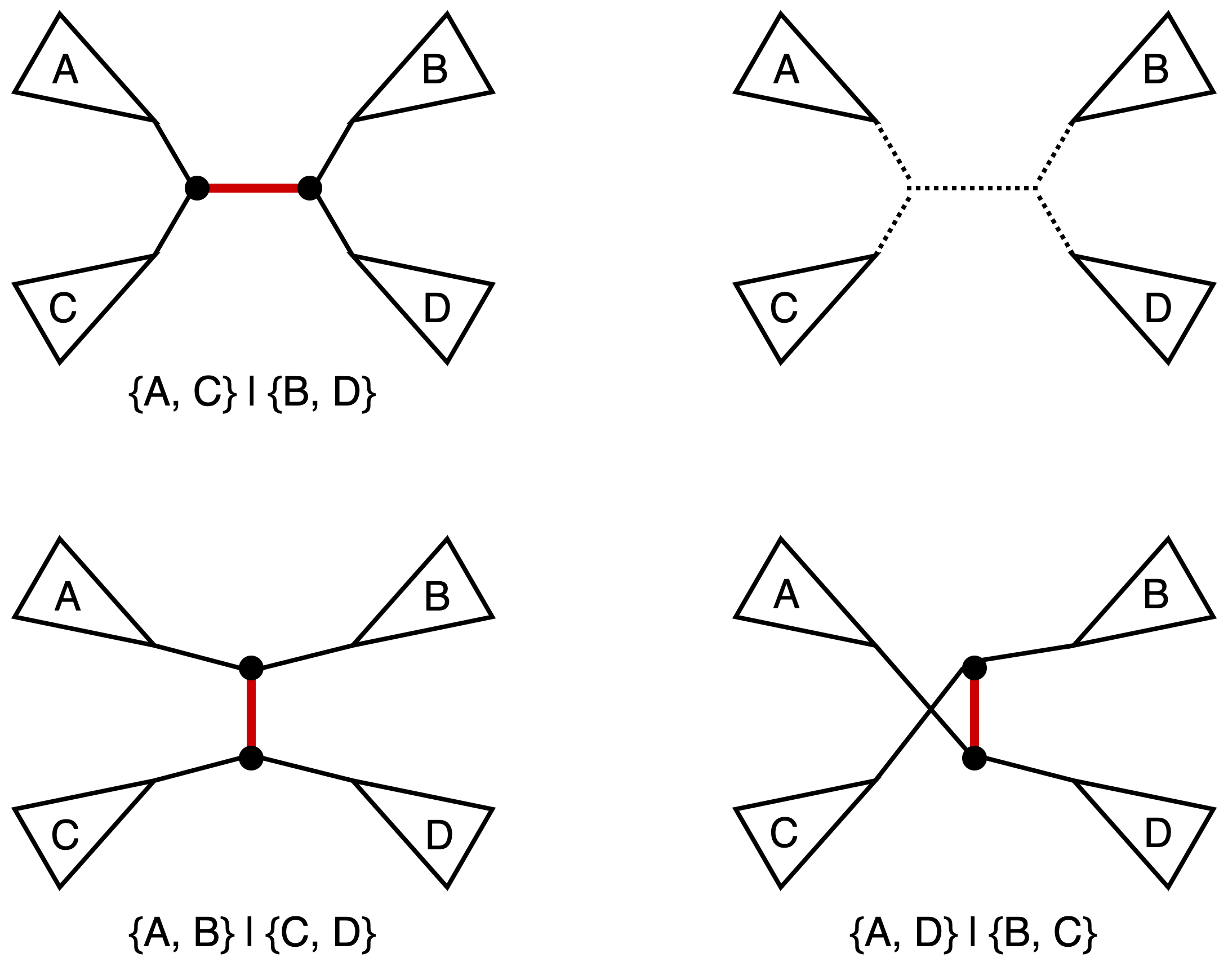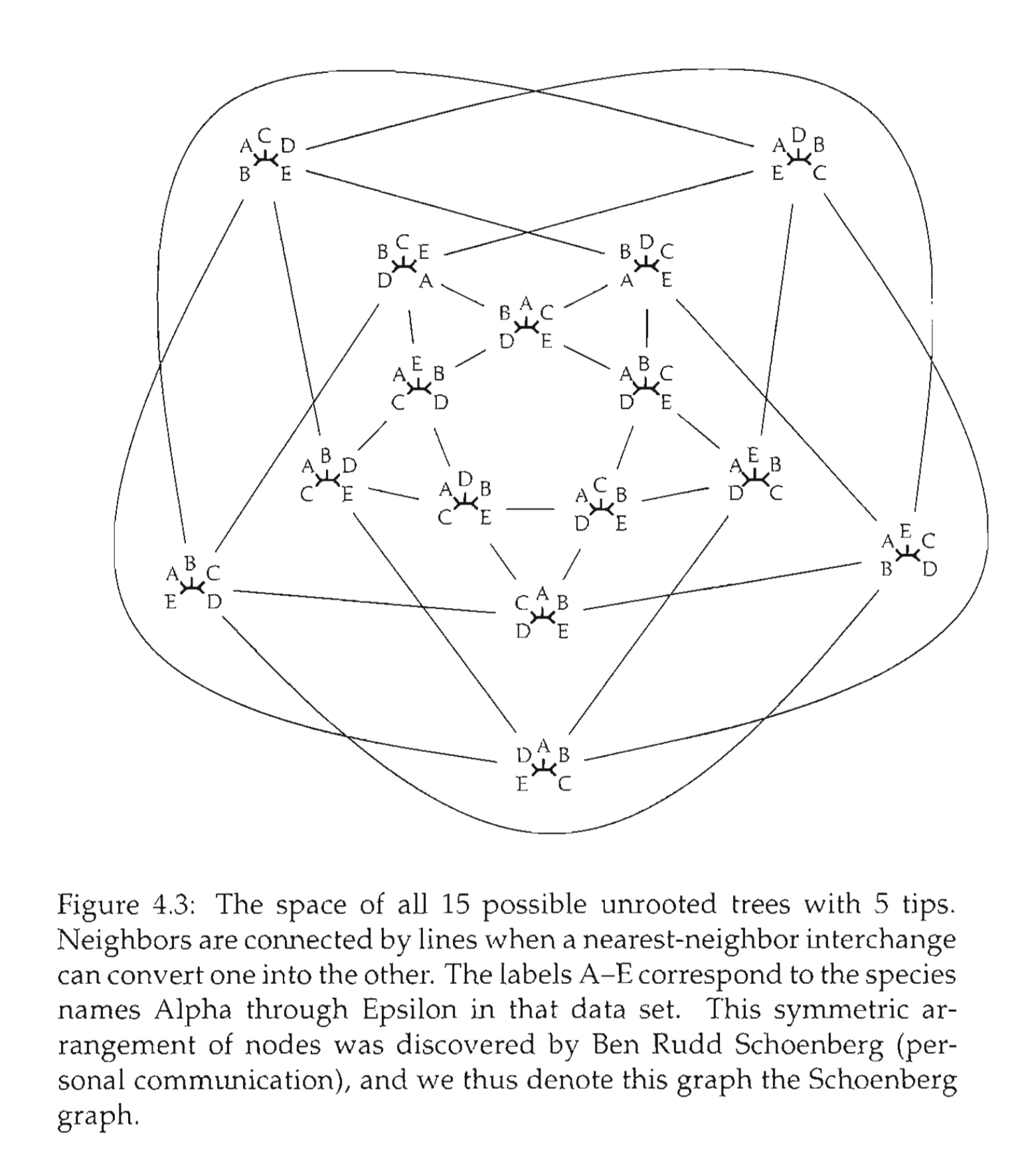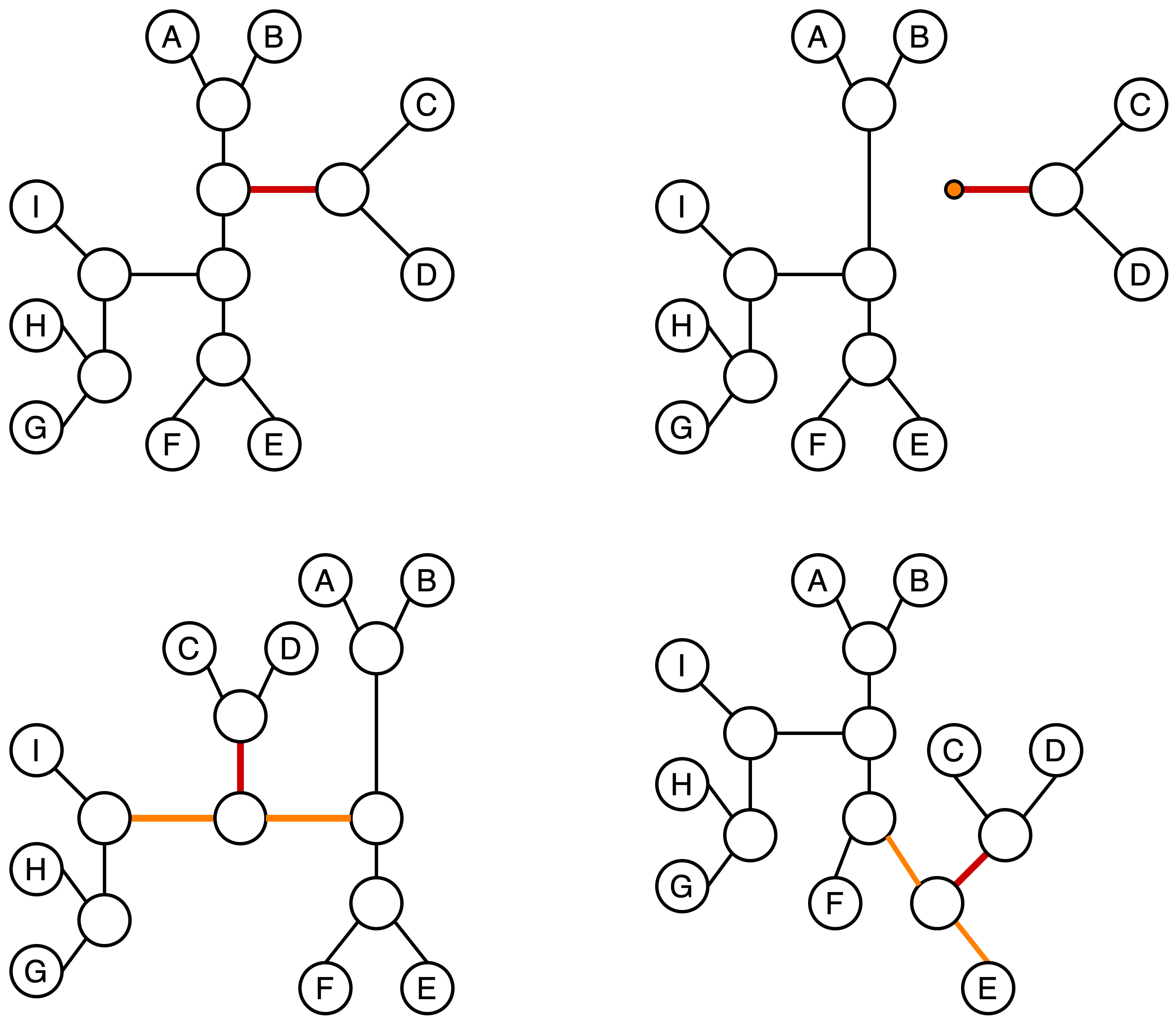# Phylogenetic Vignettes: Tree Rearrangements

As I mentioned in the previous post, I am taking some time off to figure out which direction to pursue next in the blog post series. However, that does not mean that the whole series itself is going into hiatus. In the meantime, I am going to cover a few other topics in phylogenetics. Additionally, I will cross post some of the one-off posts on Dr. Treangen’s lab website. Now, with logistics out of the way let’s get into tree rearrangements.

## Introduction

Whenever we are faced with a problem in combinatorial optimization it is often convenient (or even necessary as the majority of interesting problems turn out to be NP-hard) to come up with some heuristic methods. In particular, we often want to explore a “neighborhood” of an object and locate optimal candidates within this neighborhood after which the search can continue from the new local optima. In order to talk about neighborhoods we need to define a metric on the space of objects we are working with (we talked about metric spaces on phylogenetic trees in several previous posts, but in this case we are focusing solely on the tree topology and associated finite metric spaces). In the context of a search problem it is useful to think about the neighborhood of an object as a set of objects that can be obtained from the initial object via some fixed operation. In phylogenetics such operations on trees are typically called tree rearrangements (Felsenstein, 2004). In this post we will take a look at three classical tree rearrangement operations, but keep in mind that there are other ways to mutate trees which lead to different geometries on the tree space.

In the next three sections we will introduce in detail each of the rearrangement operations in the order of increasing neighborhood sizes considered and then briefly discuss the relationships between these three classes, as well as some implications for computational complexity of related problems.

## Nearest neighbor interchange

Nearest neighbor interchange (NNI) operation on a tree consists of picking an internal edge (i.e. an edge that is not incident to a leaf) deleting it along with the four edges incident to the vertices of the original edge, after obtaining four components as the result of the deletion there are three distinct ways of reconnecting them back out of which one yields the original tree. It is easy to see that for an unrooted bifurcating tree on n taxa (i.e. a tree with n leaves) there is a total of n-3 internal edges, and hence a total of 2(n-3) NNI-neighbors. We also note that the operation in this classical sense is defined for bifurcating trees (hence the guarantee that the NNI yields for connected components after the edge removal step). The figure below provides an example of NNI and the resulting tree neighbors.The original tree is shown in the top left corner and the marked edge is the internal edge along which we perform the NNI. The four connected components labeled A, B, C, and D are shown in the top right corner. The resulting two new trees are shown at the bottom. For each of the three trees we also include the split corresponding to the marked edge.

For a small value of n we can reasonably visualize the adjacency structure on the tree space induced by the NNI operation. Namely we can identify non-isomorphic (read: distinct from the point of view of the properties we care about) labeled tree topologies as vertices and let two vertices be connected by an edge iff we can obtain one topology from another via a single NNI.An example of adjacency graph on the space of 5 taxa unrooted bifurcating trees under the NNI operation. Original figure and caption are taken from Felsenstein, 2004, p. 40.

In general a phylogenetic tree encountered (or I guess inferred) in the wild might not be bifurcating, so it is quite natural to ask how does one generalize the notion of NNI to an arbitrary labeled unrooted tree. At the core of this operation is the process of picking a distinguished edge and then swapping components resulting from an edge deletion operation. Thus, in the case of a multifurcating tree we simply can do the same operation but with a larger set of neighbors being generated. An example is provided in the figure below.A few possible neighbors of the original multifurcating tree (top left) under the NNI on trees with varying internal node degrees. Note that in the case where we allow multifurcations we ought to be careful in the way we define the NNI operation as some interchanges can be problematic (consider what happens if we reconnect 5 components into a star graph).

There is some amount of work on the NNI operations and NNI induced metric on phylogenetic trees (Hon and Lam, 1999), although the area tends to feel relatively sparse in terms of the research done.

## Subtree prune and regraft

Subtree prune and regraft (SPR) operation on a tree consists of snipping off a branch (which can be an internal or external edge) and then inserting the snipped off subtree onto one of the edges of the remaining tree. This operation is quite well illustrated by its name, especially if you ever had to deal with grafts on actual trees (my grandfather loved to experiment with fruit tree grafting, which to his credit managed to give us a wonderful apricot half-tree on a wild apricot that used to grow in the garden). The figure below is an example of a SPR operation performed on a tree.A subtree is snipped off from the original tree along the edge marked in red (top left) and then re-attached to the remaining tree on an edge marked in orange. It can be helpful to think of this operation as temporarily turning one of the subtrees into a rooted tree and then reinserting its root into the remaining tree.

It is easy to see that SPR operation results in a larger neighborhood set than the NNI operation. It also useful to note that any tree T’ that can be obtained from a tree T via a single NNI can also be obtained via a single SPR. In other words, if we define the set NNI(T) of trees that can be obtained from T via a single NNI, and the set SPR(T) of trees that can be obtained from T via a single SPR, then the following inclusion holds: NNI(T)SPR(T) (Maddison, 1991). In the spirit of ancient geometers instead of a formalized proof, we will provide the following figure and leave it up to the reader to convince themselves that the inclusion above is indeed true.

Similarly to the NNI case we can compute the exact number of neighbors under the SPR operation for an unrooted bifurcating binary tree on n taxa. The size of this neighborhood similarly to the NNI case is independent of the topology of the tree T and is equal to 2(n-3)(2n-7) with a detailed counting argument provided by Allen and Steel, 2001 on p. 4.

We also note that the way in which we graft a subtree back in will always create an internal node of degree 3, so without a modification to this operation the question of generalizing it to multifurcating trees can be ill-posed.

## Tree bisection and reconnection

Tree bisection and reconnection (TBR) operation is the most expansive operation among the three we are considering in this post, in the sense that it yields the largest neighborhoods. TBR consists of splitting the original tree T into two subtrees T’ and T” along a branch and then reconnecting them by joining any two branches of T’ and T” via an edge (in case if one of the subtrees is just a leaf the leaf is simply joined back to the tree via a new branch; in all cases vertices might have to be added to maintain a proper bifurcating tree). The figure below illustrates a TBR operation being performed.The original tree is shown in the upper left corner. The edges to be joined are marked in orange (bottom), and the new joining edge is marked in red (bottom right). Note, that the tree obtained after the specified TBR operation is isomorphic to the tree obtained via SPR with regrafting onto the orange edge of the bottom left subtree.

The inclusion we observed for the NNI and SPR neighborhoods extends further and the following holds: NNI(T)SPR(T)TBR(T) (Maddison, 1991). Furthermore, by noting that any TBR can be thought of as a two step process where we first re-attach T” onto the specified edge of T’ and then reposition the attached T’ in order to get correct edge match, we can show that any TBR operation can be replicated by at most two successive SPR operations.

Finally, we note that unlike NNI and SPR, the TBR neighborhood size does depend on the topology of the tree T. However, and upper bound on the size of the neighborhood can be computed and is given by (2n-3)(n-3)2 (Allen and Steel, 2001, p.5).

## Induced metrics and computational (in)tractability

We already noted in the introduction that the tree rearrangement operations induce corresponding metrics on the space of phylogenetic trees (more precisely in our current case: the space of unrooted bifurcating tree topologies). We will denote these metrics dNNI(T, T’), dSPR(T, T’), and dTBR(T, T’), respectively. From the inclusion relation on the neighborhoods it immediately follows that for any two unrooted bifurcating trees we have the following inequality: dTBR(T, T’)dSPR(T, T’)dNNI(T, T’). Furthermore, from our observation in the previous section we also can conclude that dSPR(T, T’)dTBR(T, T’).

Although we have provided an explicit example of the adjacency graph under NNI metric for the space of unrooted bifurcating trees on 5 taxa, it is not immediately obvious that in the general case the NNI adjacency graph will be connected (i.e. we are not a priori guaranteed to have a connected metric space). However, it turns out that indeed the adjacency graph under the NNI metric is indeed connected, and hence the metric space induced by dNNI(T, T’) is connected. It is easy to see that as a corollary we also get connectedness for the SPR and TBR spaces.

Since the metric spaces are connected and finite we can ask what are their respective diameters (i.e. the smallest distance between two points furthest apart in the space; this is the same definition as the graph diameter). An interesting non-trivial bound on the diameter of the NNI space was derived by Li et al., 1996: authors show that the diameter of the NNI adjacency graph (which we will denote as ΔNNI) is bounded below and above by nlogn terms. More precisely, the following inequality holds:$\frac{n}{4}\log_2 n - o(n\log n)\leq \Delta_{NNI}\leq n\log_2 n + O(n)$. Allen and Steel, 2001 extend this result to the SPR and TBR cases giving the following inequalities: (a) n/2-o(n)ΔSPRn-3; (b) n/4-o(n)ΔTBRn-3.

However, knowing the diameter of a space does not imply that we can easily compute the distances within it (i.e. shortest paths via NNI, SPR or TBR operations from one tree to another). In fact, the problems of computing NNI, SPR or TBR distance between two arbitrary unrooted bifurcating trees are all NP-hard (NNI: DasGupta et al., 2000; SPR: Bordewich and Semple, 2005, Hickey et al., 2008; TBR: Hein et al., 1996). Allen and Steel, 2001 show that the SPR distance is fixed-parameter tractable, but in general the fixed-parameter tractability results in algorithms that can work efficiently only on small trees or pairs of trees which are relatively close to each other (for a more principled discussion see Whidden and Matsen, 2018). In general, it appears to be a rare case for a metric that is biologically interpretable to be efficiently computable, and vice-a-versa (take for example the Robinson-Foulds distance which is efficiently computable, but not biologically well grounded). There are some recent attempts at developing variants of tree rearrangement operations that have both biological interpretability and are computationally tractable (Collienne and Gavryushkin, 2021).

While we could possibly discuss other tree rearrangement operations or explore the discrete geometries arising from the ones we mentioned in more detail, such work would likely require multiple posts. Thus, we will wrap up here and invite the reader to continue the exploration via following the links provided in the references section.

## Data and code availability

All illustrations with the exception of the one from Felsenstein, 2004 were made with draw.io. No additional materials or code were used in this post.

## What’s next

I am still deliberating on the exact direction to take for the next stretch of posts, so in the meantime I am intending to continue the one-off posts where I pick a random, but interesting to me, topic in mathematical phylogeny and try to write a coherent text about it. As per usual, if you are interested in me writing about a specific topic do not hesitate to reach out.

## References

Allen, Benjamin L., and Mike Steel. “Subtree transfer operations and their induced metrics on evolutionary trees.” Annals of combinatorics 5, no. 1 (2001): 1-15. https://doi.org/10.1007/s00026-001-8006-8

Bordewich, Magnus, and Charles Semple. “On the computational complexity of the rooted subtree prune and regraft distance.” Annals of combinatorics 8, no. 4 (2005): 409-423. https://doi.org/10.1007/s00026-004-0229-z

Collienne, Lena, and Alex Gavryushkin. “Computing nearest neighbour interchange distances between ranked phylogenetic trees.” Journal of Mathematical Biology 82, no. 1 (2021): 1-19. https://doi.org/10.1007/s00285-021-01567-5

Felsenstein, Joseph. Inferring phylogenies. Vol. 2. Sunderland, MA: Sinauer associates, 2004.

Gupta, Bhaskar Das, Xin He, Tao Jiang, Ming Li, and John Tromp. “On computing the nearest neighbor interchange distance.” In Discrete Mathematical Problems with Medical Applications: DIMACS Workshop Discrete Mathematical Problems with Medical Applications, December 8-10, 1999, DIMACS Center, vol. 55, p. 125. American Mathematical Soc., 2000. PDF on author’s page

Hein, Jotun, Tao Jiang, Lusheng Wang, and Kaizhong Zhang. “On the complexity of comparing evolutionary trees.” Discrete Applied Mathematics 71, no. 1-3 (1996): 153-169. https://doi.org/10.1016/S0166-218X(96)00062-5

Hickey, Glenn, Frank Dehne, Andrew Rau-Chaplin, and Christian Blouin. “SPR distance computation for unrooted trees.” Evolutionary Bioinformatics 4 (2008): EBO-S419. https://doi.org/10.4137/EBO.S419

Hon, Wing-Kai, and Tak-Wah Lam. “Approximating the nearest neighbor interchange distance for evolutionary trees with non-uniform degrees.” In International Computing and Combinatorics Conference, pp. 61-70. Springer, Berlin, Heidelberg, 1999. https://doi.org/10.1007/3-540-48686-0_6

Li, Ming, John Tromp, and Louxin Zhang. “On the nearest neighbour interchange distance between evolutionary trees.” Journal of Theoretical Biology 182, no. 4 (1996): 463-467. https://doi.org/10.1006/jtbi.1996.0188

Maddison, David R. “The discovery and importance of multiple islands of most-parsimonious trees.” Systematic Biology 40, no. 3 (1991): 315-328. https://doi.org/10.1093/sysbio/40.3.315

Whidden, Chris, and Frederick A. Matsen. “Calculating the unrooted subtree prune-and-regraft distance.” IEEE/ACM transactions on computational biology and bioinformatics 16, no. 3 (2018): 898-911. https://doi.org/10.1109/TCBB.2018.2802911